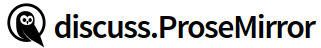# How to get index relative to the parent element

``````<table>
<tr>
<td>a</td>
<td>b</td>
</tr>
<tr>
<td>
c
</td>
<td>
d
</td>
</tr>
<tr>
<td>e</td>
<td>f</td>
</tr>
<tr>
<td>g</td>
<td>h</td>
</tr>
</table>
``````

I want to get the index of TD that I click

For example, I click cell B, and the index I want to get is 1 (cell A is 0)

i try it:

in TableTdView :

``````// tableTdNode is current click cell td
tableTrNode.forEach((item, offset, index) => {

if(item.eq(tableTdNode)){
console.log('index', index);
}

});
``````

but eq method It is only used when the textContent are not equal. If the textContent are equal, they are considered to be the same

You shouldn’t use node values like that—they don’t uniquely identify a position in the document. Use offsets whenever you need to indicate document positions, and you won’t have this problem.

If I want to insert a column after the second column, what’s the best way? I’m also studying the source code of prosemirror-tables, but I can’t understand it. Please give me some advice

Find the position, in each row, after the first column, and insert the cells there. If you have a table `table` that you know is at position `tablePos`, you could do something like

``````table.forEach((row, offset) => {
let rowPos = tablePos + 1 + offset
let insertPos = rowPos + row.firstChild.nodeSize
// Do something with insertPos
})
``````

I know this, but first of all, should I know which TD is, right? The first one is different from the third one, isn’t it?

Your code is inserted after the first TD, but what if it is inserted after the second TD